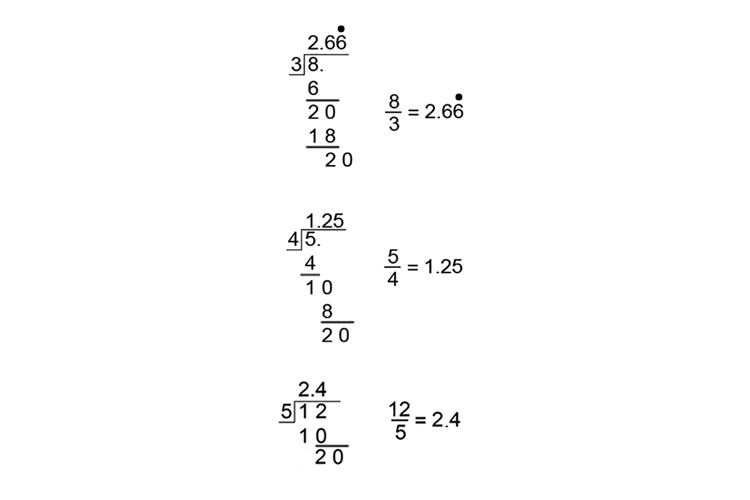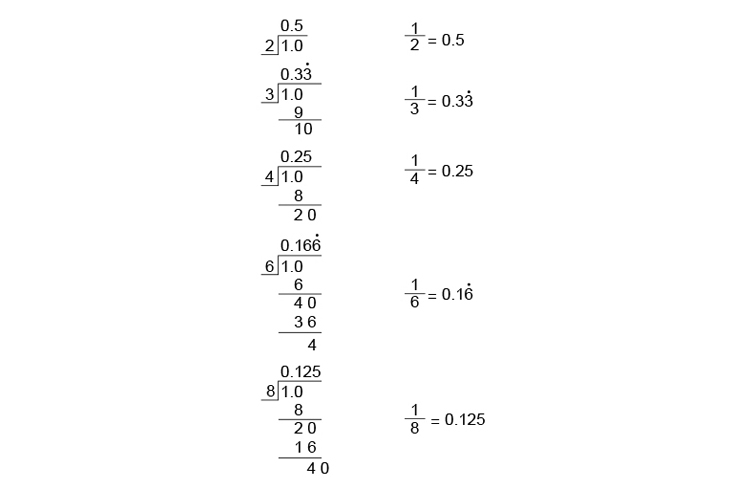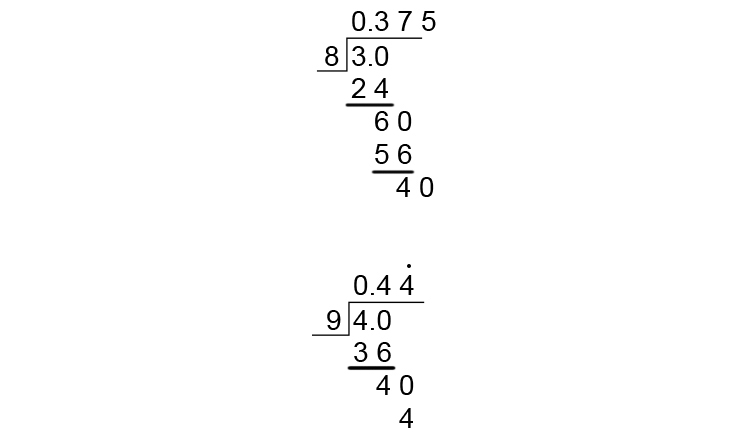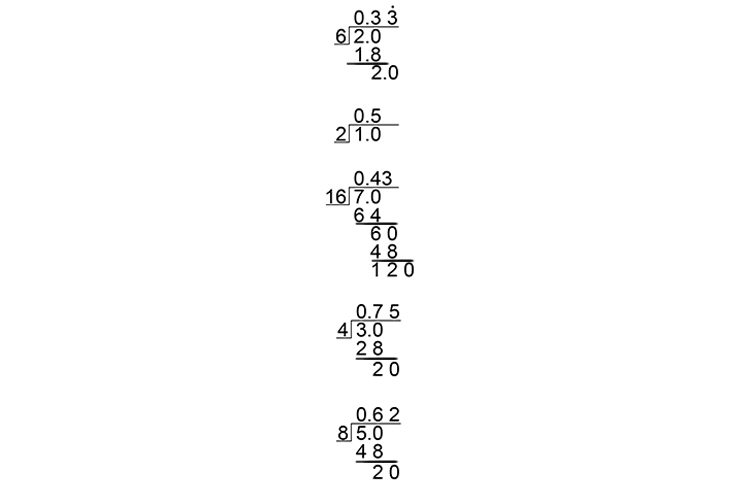# Ordering fractions by size - Method 3

Putting fractions in order smallest to largest can be achieved if you simply work out the fraction. Remember

1/2=0.5

Example 1

Put the following fractions in order of the smallest to biggest fractions.

8/3   5/4   and   12/5Now we can clearly see that

5/4  is the smallest then  12/5  then  8/3

Example 2

Put the following fractions in order of the smallest to biggest fraction

1/2   1/3   1/4   1/6   1/8

Complete the sums:Now you can clearly see that:

1/8  is the smallest followed by  1/6,  1/4  1/3  and  1/2

NOTE:

You should be familiar enough with fractions to realise that the biggest number divided into 1 would obviously be the smallest fraction.

Example 3

Which is greater 3/8  or  4/9?

Complete the sumsSo 3/8=0.375  and  4/9=0.4dot4

Clearly 4/9  is the larger fraction

Example 4

Put the following fractions in order of the smallest to biggest fraction

2/6   1/2   7/16   3/4   5/8

Complete the sums:

(Note: only go to a maximum of 2 decimal places)So 2/6=0.33   1/2=0.5   7/16=0.43   3/4=0.75   5/8=0.62

We can clearly see that the order from the smallest is:

2/6   7/16   1/2   5/8   3/4